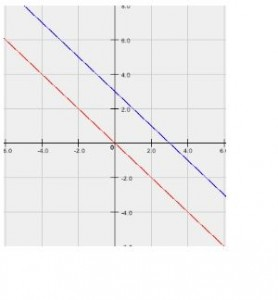# Points and Lines on the ACT and SAT

Given the coordinates of two points, you can find out many things such as

• The slope or gradient (these two words mean the same thing) of the line connecting the two points
• The equation of the line connecting the two points
• Correspondingly, the x and y-intercepts of said line
• The slope of another line that is perpendicular to the first line.### Slope of a line

Suppose we have two points with coordinates (3,8 ) and (-2, 2).  If there is a line connecting these two points, how do we find the slope of this line?

1. Subtract the y-coordinate of the second point from the first point: 8 – 2 in this case. i.e. 6
2. Subtract the x-coordinate of the second point from the first point: 3 – (-2) in this case i.e. 5
3. Divide your answer in step 1 by your answer in step 2: 6 / 5 = 1.2

In general, if you have two points with coordinates (x1, y1) and (x2, y2) then the formula for the slope is:  y1– y2/x1 – x2

### Equation of a line

The general formula for a line is y = mx + c where m is the slope of the line and c is the y-intercept (where the line cuts the y-axis).  We have already learned from the previous part how to find the slope of a line given 2 points on the line.  Here’s how we find the equation of the line.

1. Take a point with coordinates x and y e.g. the point (3,8 )) from the previous example
2. Take y – y-coordinate i.e. y – 8
3. Take x – x-coordinate i.e. x – 3
4. Multiply Step 3 by the slope, which we have found to be 1.2 earlier.  We get 1.2 (x – 3)
5. Equate Step 2 to Step 4. i.e. y – 8 = 1.2 (x – 3)
6. The equation in Step 5 is the equation of the line.  Simplify it to get y = 1.2 x + 4.4

In general, to get the equation of a line from two points, find the slope first (call it m) then plug in the values of one point into the following equation to get the equation of the line
y – y1 – m(x –x1) where m is given by the formula for slope:  y1– y2/x1 – x2

#### Intercepts

We can directly read off what the y-intercept is from the equation of the line. From the previous example, we know that the line intersects the y-axis at (0, 4.4).  To find the x-intercept, we substitute y=0 into the equation.  We then get 0 = 1.2x + 4.4  Rearranging that quickly, we realize that x = -4.4 / 1.2 which is approximately -3.67.  So the line intersects the x-axis at (0, -3.67)

### Slope of perpendicular lines

In general, if we have a line L1 and another line L2 with slopes m1 and m2 then the two lines are related by the following formula
(m1) (m2) = -1
So for the line we found in the example above, the slope of any line perpendicular to it is -1/1.2 which is -5/6 or approximately 0.83
Note that there are infinite number of lines that are perpendicular to the original line example y=1.2x + 4.4  If you are given an addition point that lines on the new perpendicular line, you will be able to find the exact equation of the perpendicular line.

Tags: